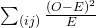The Chi-Square Distribution

# Comparison of the Chi-Square Tests

You have seen the χ2 test statistic used in three different circumstances. The following bulleted list is a summary that will help you decide which χ2 test is the appropriate one to use.

• Goodness-of-Fit: Use the goodness-of-fit test to decide whether a population with an unknown distribution “fits” a known distribution. In this case there will be a single qualitative survey question or a single outcome of an experiment from a single population. Goodness-of-Fit is typically used to see if the population is uniform (all outcomes occur with equal frequency), the population is normal, or the population is the same as another population with a known distribution. The null and alternative hypotheses are:
H0: The population fits the given distribution.
Ha: The population does not fit the given distribution.
• Independence: Use the test for independence to decide whether two variables (factors) are independent or dependent. In this case there will be two qualitative survey questions or experiments and a contingency table will be constructed. The goal is to see if the two variables are unrelated (independent) or related (dependent). The null and alternative hypotheses are:
H0: The two variables (factors) are independent.
Ha: The two variables (factors) are dependent.
• Homogeneity: Use the test for homogeneity to decide if two populations with unknown distributions have the same distribution as each other. In this case there will be a single qualitative survey question or experiment given to two different populations. The null and alternative hypotheses are:
H0: The two populations follow the same distribution.
Ha: The two populations have different distributions.

### Chapter Review

The goodness-of-fit test is typically used to determine if data fits a particular distribution. The test of independence makes use of a contingency table to determine the independence of two factors. The test for homogeneity determines whether two populations come from the same distribution, even if this distribution is unknown.

Which test do you use to decide whether an observed distribution is the same as an expected distribution?

a goodness-of-fit test

What is the null hypothesis for the type of test from (Figure)?

Which test would you use to decide whether two factors have a relationship?

a test for independence

Which test would you use to decide if two populations have the same distribution?

How are tests of independence similar to tests for homogeneity?

Answers will vary. Sample answer: Tests of independence and tests for homogeneity both calculate the test statistic the same way. In addition, all values must be greater than or equal to five.

How are tests of independence different from tests for homogeneity?

### Homework

For each word problem, use a solution sheet to solve the hypothesis test problem. Go to (Figure) for the chi-square solution sheet. Round expected frequency to two decimal places.

Is there a difference between the distribution of community college statistics students and the distribution of university statistics students in what technology they use on their homework? Of some randomly selected community college students, 43 used a computer, 102 used a calculator with built in statistics functions, and 65 used a table from the textbook. Of some randomly selected university students, 28 used a computer, 33 used a calculator with built in statistics functions, and 40 used a table from the textbook. Conduct an appropriate hypothesis test using a 0.05 level of significance.

1. H0: The distribution for technology use is the same for community college students and university students.
2. Ha: The distribution for technology use is not the same for community college students and university students.
3. 2
4. chi-square with df = 2
5. 7.05
6. p-value = 0.0294
7. Check student’s solution.
1. Alpha: 0.05
2. Decision: Reject the null hypothesis.
3. Reason for decision: p-value < alpha
4. Conclusion: There is sufficient evidence to conclude that the distribution of technology use for statistics homework is not the same for statistics students at community colleges and at universities.

Read the statement and decide whether it is true or false.

If df = 2, the chi-square distribution has a shape that reminds us of the exponential.

### Bringing It Together

1. Explain why a goodness-of-fit test and a test of independence are generally right-tailed tests.
2. If you did a left-tailed test, what would you be testing?
1. The test statistic is always positive and if the expected and observed values are not close together, the test statistic is large and the null hypothesis will be rejected.
2. Testing to see if the data fits the distribution “too well” or is too perfect.